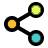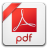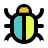Math Calculators, Lessons and Formulas

It is time to solve your math problem

mathportal.org

# Polynomial graphing calculator

This page helps you explore polynomials with degrees up to 4. The roots (x-intercepts), signs, local maxima and minima, increasing and decreasing intervals, points of inflection, and concave up-and-down intervals can all be calculated and graphed.

problem

Sketch the graph of the polynomial function

$$p(x) = 3x$$

solution

Tap the blue points to see their coordinates.

explanation

STEP 1:Find the x-intercepts

To find the x-intercepts solve, the equation $\color{blue}{ 3x = 0 }$

The solution of this equation is:

\begin{matrix}x = 0 \end{matrix}

(you can use the step-by-step polynomial equation solver to see a detailed explanation of how to solve the equation)

STEP 2:Find the y-intercepts

To find the y-intercepts, substitute $x = 0$ into $\color{blue}{ p(x) = 3x }$, so:

$$\text{Y inercept} = p(0) = 0$$

STEP 3:Find the end behavior

The end behavior of a polynomial is the same as the end behavior of a leading term.

$$\lim_{x \to -\infty} \left( 3x \right) = \lim_{x \to -\infty} 3x = \color{blue}{ -\infty }$$

The graph starts in the lower-left corner.

$$\lim_{x \to \infty} \left( 3x \right) = \lim_{x \to \infty} 3x = \color{blue}{ \infty }$$

The graph ends in the upper-right corner.

## Report an Error !

Script name : polynomial-graphing-calculator

Form values: 3x , g , Sketch the graph of polynomial 3x - mathportal

Comment (optional)

## Share Result

Or just copy and paste the link wherever you need it.Polynomial Graphing Calculator
Explore and graph polynomials.
show help ↓↓ examples ↓↓
x^2+3x-4
2x^3-3x^2-2x+3
x^4/12-x^3/3-7x^2/12+5x/6
working...
examples
example 1:ex 1:
Sketch the graph of polynomial $p(x) = x^3-2x^2-24x$
example 2:ex 2:
Find relative extrema of a function $f(x) = x^3-x$
example 3:ex 3:
Find the inflection points of $-x^4+x^2+4$
example 4:ex 4:
Sketch the graph of polynomial $p(x) = x^4-2x^2-3x+4$
Search our database of more than 200 calculators